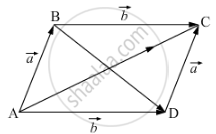Department of Pre-University Education, KarnatakaPUC Karnataka Science Class 12

# The two adjacent sides of a parallelogram are 2i-4j-5k and 2i+2j+3j Find the two unit vectors parallel to its diagonals. Using the diagonal vectors, find the area of the parallelogram. - Mathematics

Sum

The two adjacent sides of a parallelogram are 2hati-4hatj-5hatk and 2 hati+2hatj+3hatj . Find the two unit vectors parallel to its diagonals. Using the diagonal vectors, find the area of the parallelogram.

#### Solution

The two adjacent sides of a parallelogram are  2hati-4hatj-5hatk and 2 hati+2hatj+3hatj

suppose veca=2hati-4hatj-5hatk and vecb=2 hati+2hatj+3hatjThen any one diagonal of a parallelogram is vecP=veca+vecb

vecP=veca+vecb

=2hati-4hatj-5hatk + 2 hati+2hatj+3hatj

=4hati-2hatj-2hatk

Therefore, unit vector along the diagonal is vecp/|vecp|=(4hati-2hatj-2hatk)/sqrt(16+4+4)=(2hati-hatj-hatk)/sqrt(6)

Another diagonal of a parallelogram is vecP=vecb-veca

vecP=vecb-veca

=2hati+2hatj+3hatk-2hati+4hatj+5hatk

=6hatj+8hatk

Therefore, unit vector along the diagonal is vecp/|vecp|=(6hatj+8hatk)/sqrt(36+64)=(6hatj+8hatk)/sqrt(10)=(3hatj+4hatk)/5

Now

vecPxxvecP=|[hati,hatj,hatk],[4,-2,-2],[0,6,8]|

=hati(-16+12)-hatj(32-0)+hatk(24-0)

=-4hati-32hatj+24hatk

Area of parallelogram= |vecpxxvecp|/2=sqrt(16+1024+576)/2=sqrt(1616)/2=4sqrt(101)/2=2sqrt101

Concept: Geometrical Interpretation of Scalar
Is there an error in this question or solution?
Chapter 25: Vector or Cross Product - Exercise 25.1 [Page 31]

#### APPEARS IN

RD Sharma Class 12 Maths
Chapter 25 Vector or Cross Product
Exercise 25.1 | Q 36 | Page 31
Share